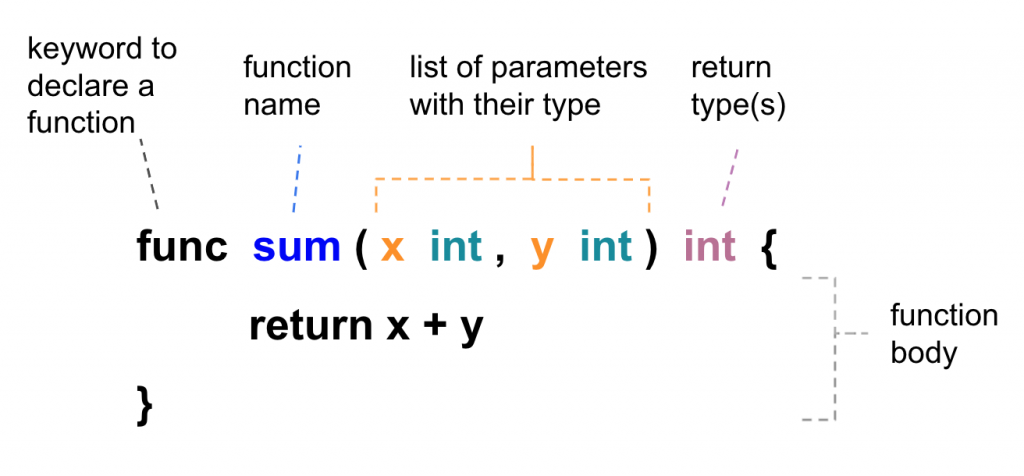#DAY 7
0
DevOps

## Declairing Functions

We can use the `func` keyword to declair a function as following:The input parameters and return type(s) are optional, such as `func main()`. Besides, a function can return multiple values as well. For example:

``````package main

import (
"errors"
"fmt"
)

func main() {
hello()

rate, _ := getRate(53, 100)
fmt.Println(rate, "%")
}

func hello() {
fmt.Println("Hello world")
}

func getRate(numerator int, denominator int) (float64, error) {
if denominator <= 0 {
err := errors.New("denominator cannot be zero or negative")
return 0.0, err
}

rate := float64(numerator) / float64(denominator) * 100
return rate, nil
}
``````

On above example, we return an `error` type value to indicate an abnormal situation and use blank identifier `_` to ignore some of the results from a function that returns multiple values.

We can put functions to another go file as well. For example:

utils.go

``````package main

import "fmt"

func hello() {
fmt.Println("Hello world")
}

``````

main.go

``````package main

func main() {
hello()
}

``````

Then you can run command "go run *.go" to test the result

``````\$ go run *.go
Hello world

``````

## Be Aware of Slices Type Parameter.

As we mention in previous blog, slice is pass by reference.
When you change the value of a slice type parameter inside the function,
The value outside the function is changed as well.

for example:

``````package main

import "fmt"

func main() {

x := int{5, 4, 3, 2, 1}
y := []int{5, 4, 3, 2, 1}
reset(x, y)
fmt.Println(x) //output = [5 4 3 2 1]
fmt.Println(y) //output = [0 4 3 2 1]
}

func reset(a int, s []int) {
a = 0
s = 0
}

``````